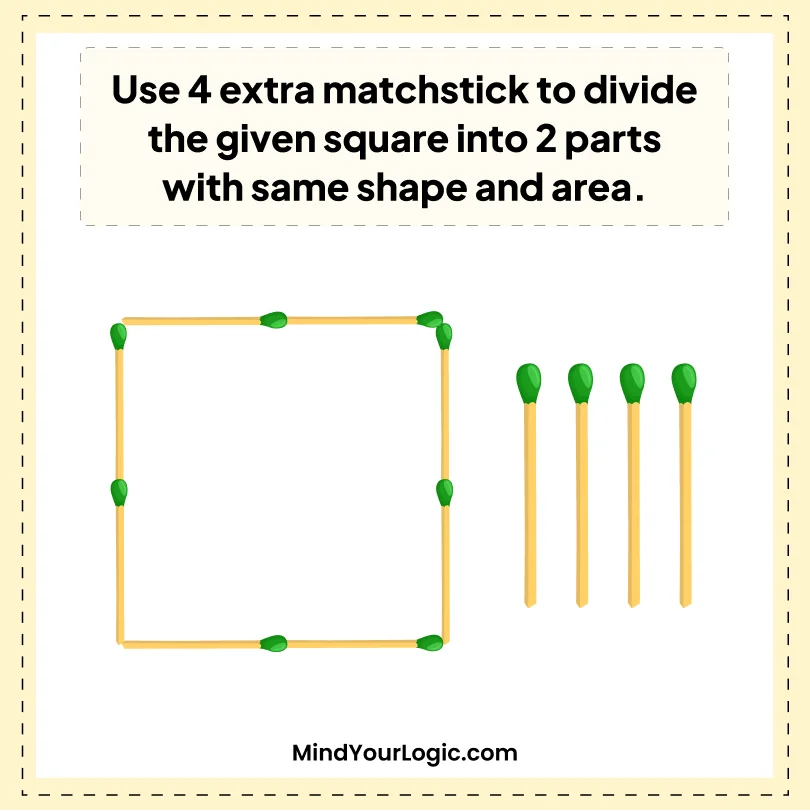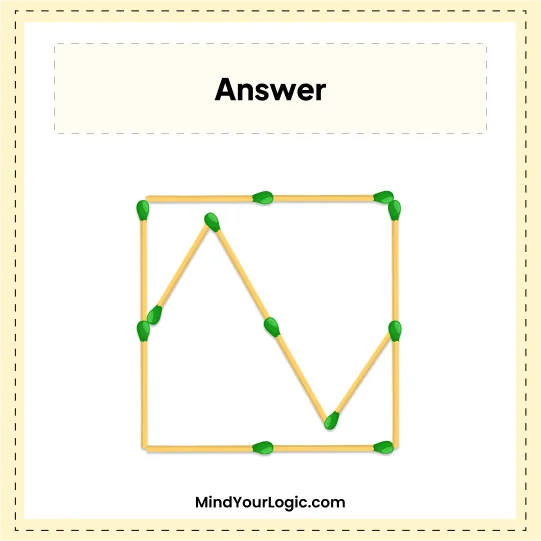# Divide the square evenly into two parts - Matchstick Puzzle

###### 41.Matchstick Puzzles
`The matchstick square is given, use an extra 4 matchsticks to divide the square evenly into two parts with the same shape and area. `•

Explanation :

``` Arrange 4 extra sticks in Alphabet ?Z? and rotate Z then place it inside the square box as given in the below image. If we cut the square into two parts, we get the square into the same shape and area as two equal parts of the square.

```